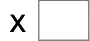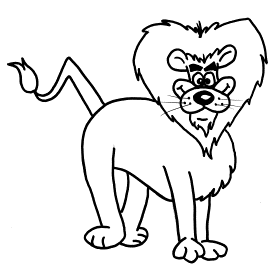9= 72   6= 608= 48   9= 9   7= 49   5= 10 7= 14   4= 28   3= 21   4= 20